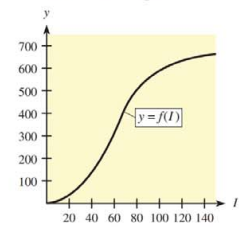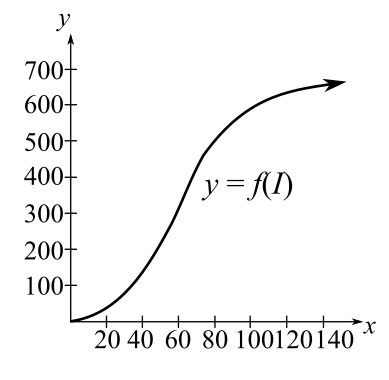Chapter 10, Problem 39RE### Mathematical Applications for the ...

11th Edition
Ronald J. Harshbarger + 1 other
ISBN: 9781305108042

#### Solutions

Chapter
Section### Mathematical Applications for the ...

11th Edition
Ronald J. Harshbarger + 1 other
ISBN: 9781305108042
Textbook Problem

# Output The following figure shows a typical graph of output y (in thousands of dollars) as a function of capital investment I (also in thousands of dollars).(a) Is the point of diminishing returns closest to the point at which 1   =   20 ,   1 − 60 ,  or  I =   120 ? Explain.(b) The average output per dollar of capital investment is defined as the total output divided by the amount of capital investment; that is,Average output = f ( I ) I Calculate the slope of a line from (0, 0) to an arbitrary point ( I , f ( I ) ) on the output graph. How is this slope related to the average output?(c) Is the maximum average output attained when the capital investment is closest to I =   40 ,  to  I =   70 ,  or to  I =   140 ? Explain.(a)

To determine

The capital investment at which the point of diminishing returns to closest among I=20, I=60, and I=120 with reason for the graph,Explanation

Given Information:

The provided graph of the capital investment and the output is shown below:

Explanation:

The critical values are the only values at which the graph can have turning points, the derivative cannot change sign anywhere except at the critical value.

This, in an interval between two critical values, the sign of the derivative at any value in the interval will be the sign of the derivative at all values in the interval.

If the first derivative of f is 0 at x0 but does not change from positive to negative or from negative to positive as x passes through x0, then the critical point at x0 is neither a relative maximum nor a relative minimum

(b)

To determine

To calculate: The slope of a line from (0,0) to an arbitrary point (I,f(I)) on the output and how the slope related to the average output if the figure shows a typical graph of output y (in thousands of dollars) as a function of capital investment I where the average output is, Average output=f(I)I..

(c)

To determine

The maximum average point attained at I=40,I=70 or I=140, when the capital investment is closest, if the figure shows a typical graph of output y (in thousands of dollars) as a function of capital investment I.### Still sussing out bartleby?

Check out a sample textbook solution.

See a sample solution

#### The Solution to Your Study Problems

Bartleby provides explanations to thousands of textbook problems written by our experts, many with advanced degrees!

Get Started

#### Evaluate the expression sin Exercises 116. (14)2

Finite Mathematics and Applied Calculus (MindTap Course List)

#### The harmonic series is: 1 + 2 + 3 + 4 + …

Study Guide for Stewart's Multivariable Calculus, 8th

#### How does a full-text database differ from other databases?

Research Methods for the Behavioral Sciences (MindTap Course List)